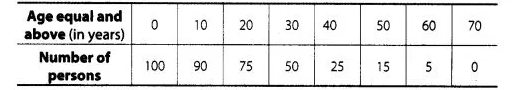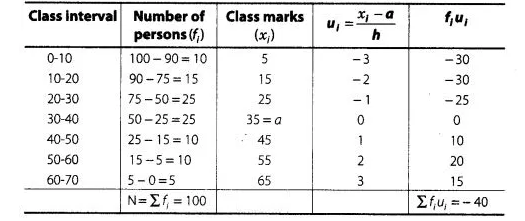# Find the mean age of 100 residents

Question:

Find the mean age of 100 residents of a town from the following data.Solution:

Here, we observe that, all 100 residents of a town have age equal and above 0. Since, 90 residents of a town have age equal and above 10.

So, 100 – 90 = 10 residents lies in the interval 0-10 and so on. Continue in this manner, we get frequency of all class intervals. Now, we construct

the frequency distribution table.Here, (assumed mean) $a=35$

and (class width) $h=10$

By step deviation method,

$\operatorname{Mean}(\bar{x})=a+\frac{\sum f_{i} u_{i}}{\sum f_{i}} \times h$

$=35+\frac{(-40)}{100} \times 10$

$=35-4=31$

Hence, the required mean age is $31 \mathrm{yr}$.